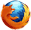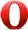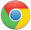# JavaScript pow() 方法JavaScript Math 对象

Math.pow(4,3);

64

## 定义和用法

pow() 方法返回 x 的 y 次幂。

## 浏览器支持Math.pow(x,y)

x 必需。底数。必须是数字。
y 必需。幂数。必须是数字。

Number x 的 y 次幂。

## 技术细节

 JavaScript 版本： 1

## 实例

var a=Math.pow(0,1);
var b=Math.pow(1,1);
var c=Math.pow(1,10);
var d=Math.pow(3,3);
var e=Math.pow(-3,3);
var f=Math.pow(2,4);

a,b,c,d,e, 和 f 输出结果：

0
1
1
27
-27
16JavaScript Math 对象